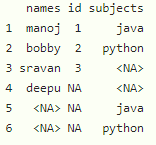GeeksforGeeks App
Open AppBrowser
Continue

# How to Remove Rows with Some or All NAs in R DataFrame?

In this article, we will discuss how to remove rows with some or all NA’s in R Programming Language.

We will consider a dataframe and then remove rows in R. Let’s create a dataframe with 3 columns and 6 rows.

## R

 `# create dataframe``data = ``data.frame``(names=``c``(``"manoj"``, ``"bobby"``, ``"sravan"``, ``"deepu"``, ``NA``, ``NA``),``                  ``id=``c``(1, 2, 3, ``NA``, ``NA``, ``NA``), ``                  ``subjects=``c``(``"java"``, ``"python"``, ``NA``, ``NA``, ``"java"``, ``"python"``))`` ` ` ` `# display``print``(data)`

Output:## Method 1: Removing Rows with Some NAs Using na.omit() Function

Here this function will remove all rows that contain NA.

Syntax:

`na.omit(dataframe)`

where, dataframe is the input dataframe.

Example:

## R

 `# create dataframe``data = ``data.frame``(names=``c``(``"manoj"``, ``"bobby"``, ``"sravan"``, ``"deepu"``, ``NA``, ``NA``),``                  ``id=``c``(1, 2, 3, ``NA``, ``NA``, ``NA``), ``                  ``subjects=``c``(``"java"``, ``"python"``, ``NA``, ``NA``, ``"java"``, ``"python"``))`` ` ` ` `# remove NA's in entire dataframe``print``(``na.omit``(data))`

Output:

```  names id subjects
1 manoj  1     java
2 bobby  2   python```

## Method 2 : Removing Rows with Some NAs Using complete.cases() Function

Here this function will remove the NAs in the dataframe.

Syntax:

`dataframe[complete.cases(dataframe), ]`

Example:

## R

 `# create dataframe``data = ``data.frame``(names=``c``(``"manoj"``, ``"bobby"``, ``"sravan"``, ``"deepu"``, ``NA``, ``NA``),``                  ``id=``c``(1, 2, 3, ``NA``, ``NA``, ``NA``), ``                  ``subjects=``c``(``"java"``, ``"python"``, ``NA``, ``NA``, ``"java"``, ``"python"``))`` ` ` ` `# remove NA's in entire dataframe``print``(data[``complete.cases``(data), ])`

Output:

```  names id subjects
1 manoj  1     java
2 bobby  2   python```

## Method 3: Removing Rows with Some NAs Using rowSums() and is.na() Functions

Here we are checking the sum of rows to 0, then we will consider the NA and then we are removing those.

Syntax:

`data[rowSums(is.na(data)) == 0, ]`

where, data is the input dataframe

Example:

## R

 `# create dataframe``data = ``data.frame``(names=``c``(``"manoj"``, ``"bobby"``, ``"sravan"``, ``"deepu"``, ``NA``, ``NA``),``                  ``id=``c``(1, 2, 3, ``NA``, ``NA``, ``NA``),``                  ``subjects=``c``(``"java"``, ``"python"``, ``NA``, ``NA``, ``"java"``, ``"python"``))`` ` `# remove NA's in entire dataframe``print``(data[``rowSums``(``is.na``(data)) == 0, ])`

Output:

```  names id subjects
1 manoj  1     java
2 bobby  2   python```

## Method 4: Removing Rows with Some NAs Using drop_na() Function of tidyr Package

Here we are going to remove the rows with NA’s using drop_na() function, Before that we have to load the tidyr library

Syntax:

`data %>% drop_na() `

where, data is the input dataframe

Example:

## R

 `# load the dplyr package``library``(``"tidyr"``)`` ` `# create dataframe``data = ``data.frame``(names=``c``(``"manoj"``, ``"bobby"``, ``"sravan"``, ``"deepu"``, ``NA``, ``NA``),``                  ``id=``c``(1, 2, 3, ``NA``, ``NA``, ``NA``),``                  ``subjects=``c``(``"java"``, ``"python"``, ``NA``, ``NA``, ``"java"``, ``"python"``))`` ` ` ` `# remove NA's in entire dataframe``print``(data % > % ``drop_na``())`

Output:

```  names id subjects
1 manoj  1     java
2 bobby  2   python```

My Personal Notes arrow_drop_up